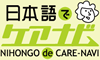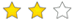kakeru

かける

multiply ; times

Example

じゅう　かける　に　わる　よん　は　ごです。

Ten multiplied by two divided by four is equal to five.

Related words

• tasu

たす

• hiku

ひく

subtract ; deduct ; minus

• waru

わる

divide